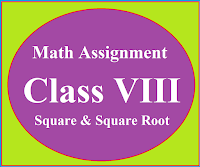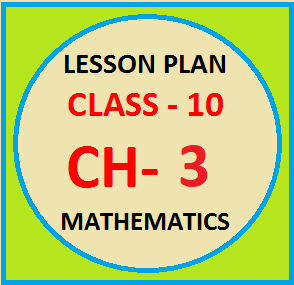## Posts

Showing posts from June, 2019

### Math Assignment Class VIII | Square & Square RootMath Assignment  Class VIII | Square & Square Root Download or Print free  assignment with answer key  for   Class  8 Squares and  Square Roots.   Important and extra questions that cover all topics of square and square root and is useful and helpful for the students. Math Assignment  Class VIII | Square & Square Root LEVEL -1

### Lesson Plan Math Class X (Ch-8) | TrigonometryE- LESSON PLAN   SUBJECT MATHEMATICS    CLASS 10 lesson plan for maths class 10 chapter 8 & 9 Trigonometry and application of trigonometry, lesson plans for mathematics teachers,  Method to write lesson plan for maths class 10,  lesson plan for maths in B.Ed. Board - CBSE CLASS –X SUBJECT- MATHEMATICS CHAPTERS  8 & 9  :- Trigonometry and applications of trigonometry TOPIC:- Chapter 8 & 9 Trigonometry and Application of Trigonometry DURATION:-    These topics are divided into seven modules and are completed in ten class meetings. PRE- REQUISITE KNOWLEDGE:- Concept of right angled triangle,  Pythagoras theorem,  Algebraic Identities. TEACHING AIDS:-    Green Board, Chalk,   Duster, Charts, smart board, laptop, projector   etc. METHODOLOGY:-   Demonstration and Lecture method. OBJECTIVES:- Introduction, and basic formulas of trigonometry. Problems based on basic formulas. Val

### Lesson Plan Math Class X (Ch- 3) | Pair of Linear Equations in Two VariablesE- LESSON PLAN    SUBJECT MATHEMATICS     CLASS 10 lesson plan for maths class 10 chapter 3  pair of linear equations in two variables, lesson plans for mathematics teachers,  Method to write lesson plan for maths class 10, lesson plan for maths in B.Ed. Board – CBSE CLASS –X SUBJECT- MATHEMATICS CHAPTER 3  :-  Pair of linear equations in two variables Lesson Plan For CBSE  Class 10 (Chapter 3) For Mathematics Teacher TOPIC:- Chapter :   03 : Pair of linear equations in two variables. DURATION:-    This topic is divided into nine modules and are completed in ten class meetings. PRE- REQUISITE KNOWLEDGE:- Chapter   4 Linear equations in two variables class 9 th Ability to solve the equations, ability to draw graphs. TEACHING AIDS:-   Green Board, Chalk,   Duster, Charts, smart board, projector, laptop   etc. METHODOLOGY:-   Demonstration and Lecture method. Lesson Plan For CBSE  Class 10 (Chapter

### Lesson Plan Math Class X (Ch-2) | PolynomialsE- LESSON PLAN   SUBJECT MATHEMATICS    CLASS 10 lesson plan for maths class 10 cbse,lesson plans for mathematics teachers,  Method to write lesson plan for maths class 10, lesson plan for maths class 10 real numbers, lesson plan for mathematics grade 10, lesson plan for maths in B.Ed. Board - CBSE CLASS –X SUBJECT- MATHEMATICS CHAPTER 2  :  Polynomials TOPIC:- Chapter:-   2: Polynomials DURATION:-    This lesson is divided into seven modules and it is completed in seven class meetings. PRE- REQUISITE KNOWLEDGE:- All definitions and important terms related to the polynomials. Polynomial Chapter 2 Class IX TEACHING AIDS:-   Green Board, Chalk,   Duster, Charts, smart board, projector and laptop   etc. METHODOLOGY:-   Demonstration and Lecture method OBJECTIVES:- Basic concepts and definitions related to the topic polynomials. Explanation of remainder and factor theorem. Re